### Home > CALC > Chapter 7 > Lesson 7.1.1 > Problem7-6

7-6.
1. Differentiate each equation with respect to x. Leave you answers in terms of only y and x. Homework Help ✎

1. y = 7ln(x + 1) − x2

2. 2x + 2y = e

3. y = etan(x)

4. (5x + 1)2 + (y + 1)2 = l

5. Find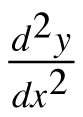for part (a). Be sure to leave all parts in terms of y and x only.

6. Find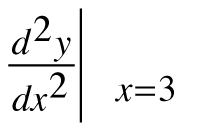for part (a).

7. Write the equation of the tangent line at x = 3 for part (a).

8. Is the tangent line an over or under approximation? Use part (f) to determine your answer.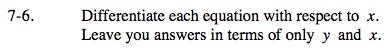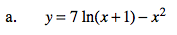$\text{The derivative of ln}(x)=\frac{1}{x}.$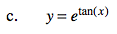Chain Rule.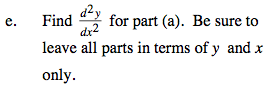$\frac{d^{2}y}{dx^{2}}=\frac{d}{dx}\text{(answer to part (a)) }.$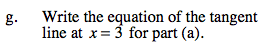The equation for the tangent line is yy(3) = y'(3)(x − 3).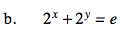Implicit Differentiation.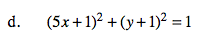$\text{Implicit differentiation. Solve for }\frac{dy}{dx}.$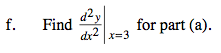Translation: Evaluate the 2nd-derivative (that you found in part (e)) at x = 3.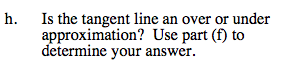Is the graph concave up or concave down?